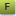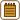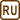## Energy of the quantum

All electromagnetic radiation is mediated by photons which are characterized by wavelength, frequency, energy and momentum.

Calculate how big the quantum of energy is for the

1. Signal from ČR1 Radiožurnál broadcasted from Prague on the frequency of 95 MHz,
2. Wave from radio navigation to the southeast of the Ruzyně airport on the frequency of 127 MHz,
3. Waves broadcasted/received by a mobile phone working on the frequency of 900 MHz,
4. Waves broadcasted/received by a mobile phone working on the frequency of 1 800 MHz,
5. Radiation in a microwave which has frequency of 2 450 MHz,
6. Signal of satellite digital broadcasting channel ČT1 on the frequency of 12 382 GHz.

For all the cases determine the corresponding wavelength.

• #### Notation

 f1 = 95 MHz broadcasting frequency ČR1 Radiožurnál f2 = 127 MHz radio navigation frequency near Prague airport f3 = 900 MHz signal frequency from mobile phone f4 = 1 800 MHz signal frequency from mobile phone f5 = 2 450 MHz radiation frequency in microwave f6 = 12 382 MHz frequency of satellite digital broadcasting channel ČT1 λi = ? (m) wavelength of i-th radiation source Ei = ? (eV) energy of i-th radiation source

From tables:

 h = 6.6·10−34 J s Planck’s constant in SI units h = 4.1·10−15 eV s Planck’s constant in eV s units c = 3.0·108 m s−1 speed of light
• #### Hint

Energy of a photon is proportionate to the frequency of the corresponding wavelength.

Wavelength is inversely proportional to the photon’s frequency.

You can read more about properties of photon here.

• #### Solution

Photon has zero rest mass and moves with speed of light. Photon’s overall energy is proportional to the frequency f of given wavelength

$E = hf ,$

where h is the Planck’s constant.

We can determine the corresponding wavelength of electromagnetic radiation with the following formula

$\lambda = {c \over f},$

where c is the speed of light in vacuum.

Now we can easily determine the characteristics of given wavelengths:

 f λ E 1. 95 MHz 3.16 m 0.4 μeV 2. 127 MHz 2.36 m 0.5 μeV 3. 900 MHz 0.33 m 3.7 μeV 4. 1 800 MHz 0.17 m 7.4 μeV 5. 2 450 MHz 0.12 m 10.1 μeV 6. 12 382 MHz 0.02 m 51.2 μeV